# Momentum

In classical mechanics, the momentum (SI unit kg m/s) of an object is the product of the mass and velocity of the object. Conceptually, the momentum of a moving object can be thought of as how difficult it would be to stop the object. As such, it is a natural consequence of Newton's first and second laws of motion. Having a lower speed or having less mass (how we measure inertia) results in having less momentum.

Momentum is a conserved quantity, meaning that the total momentum of any closed system (one not affected by external forces, and whose internal forces are not dissipative as heat or light) cannot be changed.

The concept of momentum in classical mechanics was originated by a number of great thinkers and experimentalists. René Descartes referred to mass times velocity as the fundamental force of motion. Galileo in his Two New Sciences used the term "impeto" (Italian), while Newton's Laws of Motion uses motus (Latin), which has been interpreted by subsequent scholars to mean momentum. (For accurate measures of momentum, see the section "modern definitions of momentum" on this page.)

## Momentum in Newtonian mechanics

If an object is moving in any reference frame, then it has momentum in that frame. It is important to note that momentum is frame dependent. That is, the same object may have a certain momentum in one frame of reference, but a different amount in another frame. For example, a moving object has momentum in a reference frame fixed to a spot on the ground, while at the same time having zero momentum in a reference frame that is moving along with the object.

The amount of momentum that an object has depends on two physical quantities—the mass and the velocity of the moving object in the frame of reference. In physics, the symbol for momentum is usually denoted by a small bold p (bold because it is a vector); so this can be written: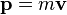$\mathbf{p}= m \mathbf{v}$

where:

p is the momentum
m is the mass
v the velocity

(using bold text for vectors).

The origin of the use of p for momentum is unclear. It has been suggested that, since m had already been used for "mass," the p may be derived from the Latin petere ("to go") or from "progress" (a term used by Leibniz).

The velocity of an object at a particular instant is given by its speed and the direction of its motion at that instant. Because momentum depends on and includes the physical quantity of velocity, it too has a magnitude and a direction and is a vector quantity. For example, the momentum of a five-kg bowling ball would have to be described by the statement that it was moving westward at two m/s. It is insufficient to say that the ball has ten kg m/s of momentum because momentum is not fully described unless its direction is also given.

## Momentum for a system

### Relating to mass and velocity

The momentum of a system of objects is the vector sum of the momenta of all the individual objects in the system.$\mathbf{p}= \sum_{i = 1}^n m_i \vec\mathbf{v}_i = m_1 \vec\mathbf{v}_1 + m_2 \vec\mathbf{v}_2 + m_3 \vec\mathbf{v}_3 + ... + m_n \vec\mathbf{v}_n$

where$\mathbf{p}$ is the momentum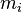$m_i$ is the mass of object i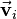$\vec\mathbf{v}_i$ the vector velocity of object i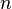$n\$ is the number of objects in the system

### Relating to force

Force is equal to the rate of change of momentum: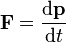$\mathbf{F} = {\mathrm{d}\mathbf{p} \over \mathrm{d}t}$.

In the case of constant mass and velocities much less than the speed of light, this definition results in the equation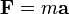$\mathbf{F} = m\mathbf{a}$—commonly known as Newton's second law.

If a system is in equilibrium, then the change in momentum with respect to time is equal to zero: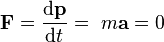$\mathbf{F} = {\mathrm{d}\mathbf{p} \over \mathrm{d}t}=\ m\mathbf{a}= 0$

## Conservation of momentum

The principle of conservation of momentum states that the total momentum of a closed system of objects (which has no interactions with external agents) is constant. One of the consequences of this is that the center of mass of any system of objects will always continue with the same velocity unless acted on by a force outside the system.

In an isolated system (one where external forces are absent) the total momentum will be constant—this is implied by Newton's first law of motion. Newton's third law of motion, the law of reciprocal actions, which dictates that the forces acting between systems are equal in magnitude, but opposite in sign, is due to the conservation of momentum.

Since momentum is a vector quantity it has direction. Thus, when a gun is fired, although overall movement has increased compared to before the shot was fired, the momentum of the bullet in one direction is equal in magnitude, but opposite in sign, to the momentum of the gun in the other direction. These then sum to zero which is equal to the zero momentum that was present before either the gun or the bullet was moving.

### Collisions

Momentum has the special property that, in a closed system, it is always conserved, even in collisions. Kinetic energy, on the other hand, is not conserved in collisions if they are inelastic (where two objects collide and move off together at the same velocity). Since momentum is conserved it can be used to calculate unknown velocities following a collision.

A common problem in physics that requires the use of this fact is the collision of two particles. Since momentum is always conserved, the sum of the momenta before the collision must equal the sum of the momenta after the collision: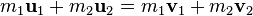$m_1 \mathbf u_{1} + m_2 \mathbf u_{2} = m_1 \mathbf v_{1} + m_2 \mathbf v_{2} \,$

where:

u signifies vector velocity before the collision
v signifies vector velocity after the collision.

Usually, we either only know the velocities before or after a collision and would like to also find out the opposite. Correctly solving this problem means you have to know what kind of collision took place. There are two basic kinds of collisions, both of which conserve momentum:

• Elastic collisions conserve kinetic energy as well as total momentum before and after collision.
• Inelastic collisions don't conserve kinetic energy, but total momentum before and after collision is conserved.

#### Elastic collisions

A collision between two pool balls is a good example of an almost totally elastic collision. In addition to momentum being conserved when the two balls collide, the sum of kinetic energy before a collision must equal the sum of kinetic energy after: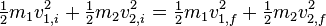$\begin{matrix}\frac{1}{2}\end{matrix} m_1 v_{1,i}^2 + \begin{matrix}\frac{1}{2}\end{matrix} m_2 v_{2,i}^2 = \begin{matrix}\frac{1}{2}\end{matrix} m_1 v_{1,f}^2 + \begin{matrix}\frac{1}{2}\end{matrix} m_2 v_{2,f}^2 \,$

Since the one-half factor is common to all the terms, it can be taken out right away.

In the case of two objects colliding head on we find that the final velocity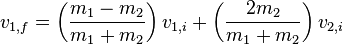$v_{1,f} = \left( \frac{m_1 - m_2}{m_1 + m_2} \right) v_{1,i} + \left( \frac{2 m_2}{m_1 + m_2} \right) v_{2,i} \,$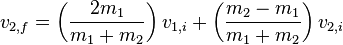$v_{2,f} = \left( \frac{2 m_1}{m_1 + m_2} \right) v_{1,i} + \left( \frac{m_2 - m_1}{m_1 + m_2} \right) v_{2,i} \,$

which can then easily be rearranged to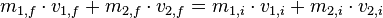$m_{1,f} \cdot v_{1,f} + m_{2,f} \cdot v_{2,f} = m_{1,i} \cdot v_{1,i} + m_{2,i} \cdot v_{2,i}\,$

Special Case: m1 much greater than m2
Now consider if [[mass] ] of one body say m1 is far more than m2 (m1>>m2). In that case m1+m2 is approximately equal to m1. And m1-m2 is approximately equal to m1.

Put these values in the above equation to calculate the value of v2 after collision. The expression changes to v2 final is 2*v1-v2. Its physical interpretation is in case of collision between two body one of which is very heavy, the lighter body moves with twice the velocity of the heavier body less its actual velocity but in opposite direction.

Special Case: m1 equal to m2
Another special case is when the collision is between two bodies of equal mass. Say body m1 moving at velocity v1 strikes body m2 that is at rest (v2). Putting this case in the equation derived above we will see that after the collision, the body that was moving (m1) will start moving with velocity v2 and the mass m2 will start moving with velocity v1. So there will be an exchange of velocities.
Now suppose one of the masses, say m2, was at rest. In that case after the collision the moving body, m1, will come to rest and the body that was at rest, m2, will start moving with the velocity that m1 had before the collision.
Please note that all of these observations are for an elastic collision.
This phenomenon called “Newton's cradle,” one of the most well-known examples of conservation of momentum, is a real life example of this special case.

##### Multi-dimensional collisions

In the case of objects colliding in more than one dimension, as in oblique collisions, the velocity is resolved into orthogonal components with one component perpendicular to the plane of collision and the other component or components in the plane of collision. The velocity components in the plane of collision remain unchanged, while the velocity perpendicular to the plane of collision is calculated in the same way as the one-dimensional case.

For example, in a two-dimensional collision, the momenta can be resolved into x and y components. We can then calculate each component separately, and combine them to produce a vector result. The magnitude of this vector is the final momentum of the isolated system.

#### Inelastic collisions

A common example of a perfectly inelastic collision is when two snowballs collide and then stick together afterwards. This equation describes the conservation of momentum:$m_1 \mathbf v_{1,i} + m_2 \mathbf v_{2,i} = \left( m_1 + m_2 \right) \mathbf v_f \,$

It can be shown that a perfectly inelastic collision is one in which the maximum amount of kinetic energy is converted into other forms. For instance, if both objects stick together after the collision and move with a final common velocity, one can always find a reference frame in which the objects are brought to rest by the collision and 100 percent of the kinetic energy is converted.

## Momentum in relativistic mechanics

In relativistic mechanics, momentum is defined as: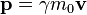$\mathbf{p} = \gamma m_0\mathbf{v}$

where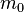$m_0\,$ is the mass of the object moving,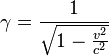$\gamma = \frac{1}{\sqrt{1 - \frac{v^2}{c^2}}}$ is the Lorentz factor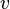$v\,$ is the relative velocity between an object and an observer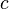$c\,$ is the speed of light.

Relativistic momentum becomes Newtonian momentum at low speeds.

Momentum of massless objects

Massless objects such as photons also carry momentum. The formula is: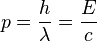$p = \frac{h}{\lambda} = \frac{E}{c}$

where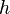$h\,$ is Planck's constant,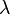$\lambda\,$ is the wavelength of the photon,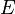$E\,$ is the energy the photon carries and$c\,$ is the speed of light.

### Momentum in electromagnetism

When electric and/or magnetic fields move, they carry momentum. Light (visible light, UV, radio) is an electromagnetic wave and also has momentum. Even though photons (the particle aspect of light) have no mass, they still carry momentum. This leads to applications such as the solar sail.

Momentum is conserved in an electrodynamic system (it may change from momentum in the fields to mechanical momentum of moving parts).